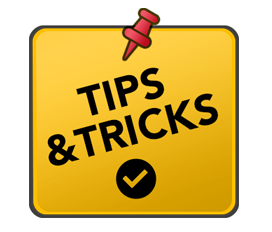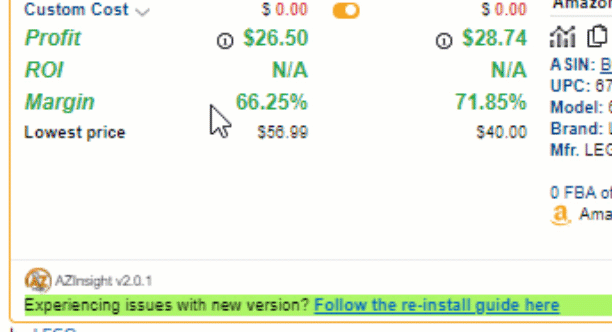# Tips & Tricks # 21 - Performing on the fly calculations using the auto-calculate for buy or sell priceWhat we're trying to do: Perform basic arithmetic calculations on the fly on the Buy or Sell price.

Scenario: Let's assume in this case you know you can get a product 50% off from the MSRP (Manufacturer's Suggested Retail Price). To this hover, your mouse over the Lowest price and note the MSRP. Then go to the Buy Cost Input field under FBA or FBM and type in 49.99*.50. The 49.99 is the MSRP, and .50 is the percent in the decimal format of percent off.

Note: This can be done both on the Buy Cost or Sell Price inputs.

The input field accepts the following arithmetic functions:  * = muliplication, / = division, + = addition, - = subtraction